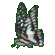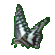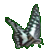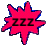Volume (Capacity) of Pyramids & Cones    Game Tips:

- In this game, the Volume equals one third times base Area times Height. Use V=(1/3)(AH).
The diagrams shown are not drawn to scale size.

- When a pyramid has a rectangular base, then the base Area equals Length times Width. Use A=LW.

- For a cone, the circular base Area equals (Pi) times Radius times Radius. Use A=(Pi)(R2).
The value of 'Pi' or 'π' is approximately '3.14' .

- The volume of a cylinder is V = πR2H.
- The volume of a cone is V = (1/3)πR2H.

- Your Game Score is reduced by the number of butterfly hits.

- To slow the game speed repeat tap/click on the word Slider.
- To increase the game speed repeat tap/click on the word Math.
- Speed can also be adjusted with a keyboard's - and + keys.

- Refresh/Reload the web page to restart the game.